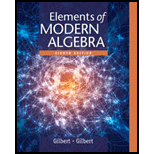# Exercises For the given permutations, f and h , find a permutation g such that h is the conjugate of f by g –that is, such that h = g f g − 1 . a. f = ( 1 , 5 , 9 ) ; h = ( 2 , 6 , 4 ) b. f = ( 1 , 3 , 5 , 7 ) ; h = ( 3 , 4 , 6 , 8 ) c. f = ( 1 , 3 , 5 ) ( 2 , 4 ) ; h = ( 2 , 4 , 3 ) ( 1 , 5 ) d. f = ( 1 , 2 , 3 ) ( 4 , 5 ) ; h = ( 2 , 3 , 4 ) ( 1 , 6 ) e. f = ( 1 , 4 , 7 ) ( 2 , 5 , 8 ) ; h = ( 1 , 5 , 4 ) ( 2 , 3 , 6 ) f. f = ( 1 , 3 , 5 ) ( 2 , 4 , 6 ) ; h = ( 1 , 2 , 4 ) ( 3 , 5 , 6 )### Elements Of Modern Algebra

8th Edition
Gilbert + 2 others
Publisher: Cengage Learning,
ISBN: 9781285463230### Elements Of Modern Algebra

8th Edition
Gilbert + 2 others
Publisher: Cengage Learning,
ISBN: 9781285463230

#### Solutions

Chapter 4.1, Problem 13E
Textbook Problem

## Expert Solution

### Want to see the full answer?

Check out a sample textbook solution.

### Want to see this answer and more?

Experts are waiting 24/7 to provide step-by-step solutions in as fast as 30 minutes!*

*Response times vary by subject and question complexity. Median response time is 34 minutes and may be longer for new subjects.